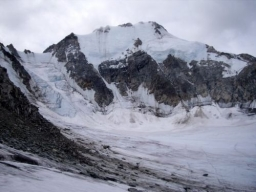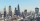# The temperature 5

The temperature at 4pm was 53° Fahrenheit the temperature dropped 3° each hour from 4pm to 9pm which equation below accurately represents the temperature at 9pm

t2 =  37 °F

### Step-by-step explanation:Did you find an error or inaccuracy? Feel free to write us. Thank you!## Related math problems and questions:

• TrianglesFind out whether given sizes of the angles can be interior angles of a triangle: a) 23°10',84°30',72°20' b) 90°,41°33',48°37' c) 14°51',90°,75°49' d) 58°58',59°59',60°3'
• Temperature differenceLibya's highest temperature was recorded at 58 degrees Celsius, and the lowest was recorded at -88 degrees Celsius. What is the temperature difference?
• If the 3If the temperature of a city drops 2° everyday and increases by 3° on every 5 days, how long does it take to drop to -15° from temperature +5°?
• Temperature 5Temperature outside starts at 0 Fahrenheit. Over time the temperature changes at a rate of -0.6 Fahrenheit per hour. How long will it take for the temperature to reach -4.5 Fahrenheit?
• Temperature increaseIf the temperature at 9:am is 50 degrees. What is the temperature at 5:00pm if the temperature increases 4 degrees Fahrenheit each hour?
• On SundayOn Sunday the temperature reached a high of 38° above 0. That night it dropped to 23° below 0. What is the difference between the high and low temperatures for Sunday?
• Equal temperatureThe temperatures of the two cities were measured at the same time. The temperature in city A was 60 degrees And rose at a constant rate of 2 degrees per hour. The temperature in city B was 40° and rose at a constant rate of 10° per hour Enter the time in
• Gamma angleFind the magnitude of the gamma angle in triangle ABC if: α = 38° 56 ’and β = 47° 54’.
• The temperature 4The temperature was very cold, then it doubled, then it dropped 10 degrees, and then it increased by 40 degrees. The temperature is now 16 degrees. What was the starting temperature?
• Degrees FahrenheitC= (5)/(9)(F−32) The equation above shows how temperature F, measured in degrees Fahrenheit, relates to a temperature C, measured in degrees Celsius. Based on the equation, which of the following must be true? I. A temperature increase of 1 degree Fahrenh
• Temperature change 3At 2 PM, The temperature was 76 degrees Fahrenheit. At 8 PM, the temperature was 58 degrees Fahrenheit. What was the change in temperature?
• The temperature 3The temperature is -12 degrees Fahrenheit. During the following 5 hours, the temperature decrease by 23 degrees Fahrenheit. What is the weather at 11 pm?
• Outside temperatureThe temperature outside was 57 degree Fahrenheit. During the next few hours it decreased by 18 degrees and then increased by 23 degrees. Find new temperature.
• Mixing Celsius and FahrenheitAdd up three temperatures: 5°F +6°F +0°C
• AnglesThe triangle is one outer angle 158°54' and one internal angle 148°. Calculate the other internal angles of a triangle.
• NoontimeIn the morning temperature was 110°F. By noontime, it has gone up by 15°F. What was the noon temperature? (Give your answer in °F)
• Temperature rise and fallOn Friday, the temperature was 82°F. The temperature changed by –2°F on Saturday, and then it changed by 5°F on Sunday. What was the temperature on Sunday? How did the temperature change? Note we consider the mathematical problem formulated in this way to